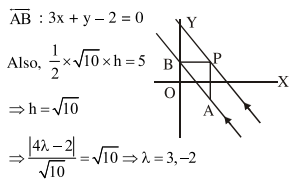# Solve this following

Question:

Let two points be $\mathrm{A}(1,-1)$ and $\mathrm{B}(0,2)$. If a point $\mathrm{P}\left(\mathrm{x}^{\prime}, \mathrm{y}^{\prime}\right)$ be such that the area of $\triangle \mathrm{PAB}=5 \mathrm{sq}$. units and it lies on the line, $3 x+y-4 \lambda=0$, then a value of $\lambda$ is

1. 1

2. 4

3. 3

4. $-3$

Correct Option: , 3

Solution: